### 13.4.2.1 Conversion to a phase transition equation

The final step is to convert the equations into phase space form. A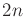-dimensional phase vector is introduced as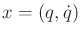. The task is to obtain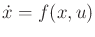, which representsscalar equations. The firstequations are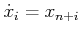forfromto. The finalequations are obtained by solving for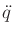.

Suppose that the general form in (13.142) is used. Solving foryields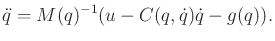(13.148)

The phase variables are then substituted in a straightforward manner. Each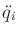forfromtobecomes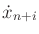, and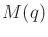,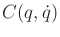, and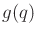are expressed in terms of. This completes the specification of the phase transition equation.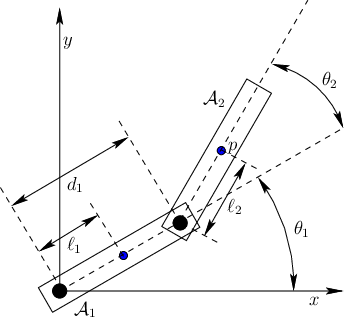Figure 13.13 shows a two-link manipulator for which there are two revolute joints and two links,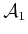and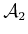. Hence,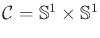. Let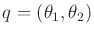denote a configuration. Each of the two joints is controlled by a motor that applies a torque. Let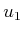apply to the base, and let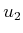apply to the joint betweenand. Let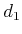be the link length of. Let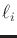be the distance from the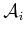origin to its center of mass. For each, let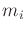and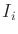be its mass and moment of inertia, respectively.

The kinetic energy ofis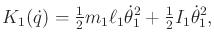(13.149)

and the potential energy ofis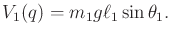(13.150)

The kinetic energy ofis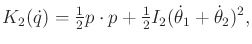(13.151)

in which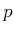denotes the position of the center of mass ofand is given from (3.53) as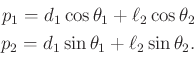(13.152)

The potential energy ofis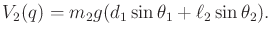(13.153)

At this point, the Lagrangian function can be formed as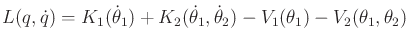(13.154)

and inserted into (13.118) to obtain the differential constraints in implicit form, expressed in terms of,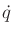, and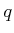. Conversion to the phase space is performed by solving the implicit constraints forand assigning, in whichis a four-dimensional phase vector.

Rather than performing the computations directly using (13.118), the constraints can be directly determined using (13.140). The terms are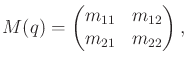(13.155)

in which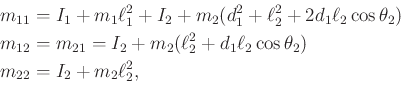(13.156)

and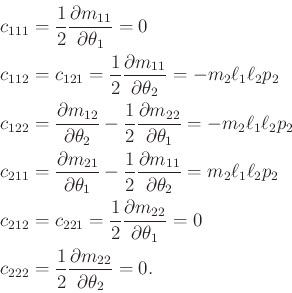(13.157)

The final term is defined as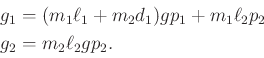(13.158)

The dynamics can alternatively be expressed using,, andin (13.142). The Coriolis matrix is defined using (13.143) to obtain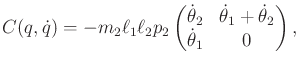(13.159)

in which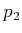is defined in (13.152) and is a function of. For convenience, let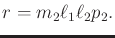(13.160)

The resulting expression, which is now a special form of (13.142), is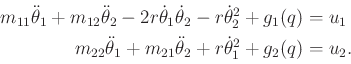(13.161)

The phase transition equation is obtained by letting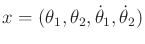and substituting the state variables into (13.161). The variables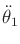and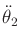become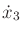and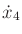, respectively. The equations must be solved forand. An extension of this model to motors that have gear ratios and nonnegligible mass appears in .The example provided here barely scratches the surface on the possible systems that can be elegantly modeled. Many robotics texts cover cases in which there are more links, different kinds of joints, and frictional forces [366,725,856,907,994].

The phase transition equation for chains of bodies could alternatively be derived using the Newton-Euler formulation of mechanics. Even though the Lagrangian form is more elegant, the Newton-Euler equations, when expressed recursively, are far more efficient for simulations of multibody dynamical systems [366,863,994].

Steven M LaValle 2020-08-14